# MCQs on Electrical And Electronics Measurements

##### Page 17 of 19. Go to page 1 2 3 4 5 6 7 8 9 10 11 12 13 14 15 16 17 18 19
01․ Consider the following statements : In a measuring instrument, 1. Linearity is more important than sensitivity. 2. High precision indicates high accuracy 3. Accuracy cannot be better than resolution. Of these statements
1 and 3 are correct
1 and 2 are correct
1, 2 and 3 are correct
2 and 3 are correct

Linearity is more important than sensitivity so that output can vary linearly with input. High precision do not indicate high accuracy. Resolution is the smallest output that can be displayed on the instrument clearly. So if resolution is high then instrument holds accuracy.

02․ A resistance is measured by the voltmeter-ammeter method employing dc excitation and a voltmeter of very high resistance connected directly across the unknown resistance. If the voltmeter and ammeter reading are subject to maximum possible errors of ± 2.4 % and ± 1.0 % respectively, then the magnitude of the maximum possible percentage error in the value of resistance deduced from the measurement is nearly
± 1%
± 2.4%
± 3.4%
± 1.4%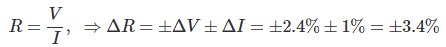03․ The limiting errors of measurement of power consumed by and the current passing through a resistance are ±1.5% and ±1% respectively. What is the limiting error ( in %) of measurement of resistance ?
± 1.5%
± 1%
± 2.5%
±3.5%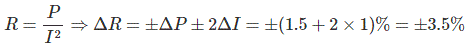04․ The expected value of the voltage across a resistor is 80 V, and the measured value is 79 V. What is the percentage error and relative accuracy of the measurement ?
1.25% & 98.75%
1.5% & 99%
1.25% & 98.25%
1.5% & 98.75%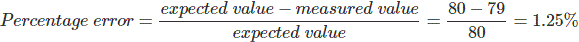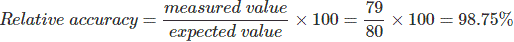05․ A voltage of 2.70 V is being measured by an analog indicating instrument having a scale range of 0 – 5.0 V. If the instrument reads 2.65 V, then absolute error in measurement is
0.01V
0.05V
0.01V
0.25V

Absolute error = Actual value - Measured value = 2.70 - 2.65 = 0.05 V

06․ The current ‘I’ through a resistance R is measures with the following uncertainties I = 4 A ± 0.5%, R = 100Ω±0.2%. If power is computed from these two measured quantities, the uncertainty in the power computed will be
1%
0.5%
1.5%
1.2%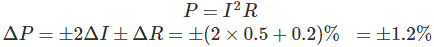07․ A set of independent current measurements taken by four observers was recorded as : 117.02 mA, 117.11 mA, 117.08 mA and 117.03 mA. What is the range of error ?
± 0.045
± 0.054
± 0.065
± 0.056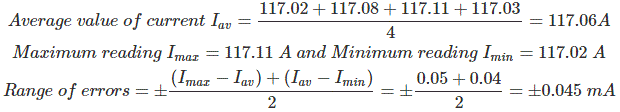08․ 0-150 V voltmeter has an accuracy of 1% of full-scale reading. The voltage measured by the instrument is 75 V. The limiting error is
1%
2%
3%
4%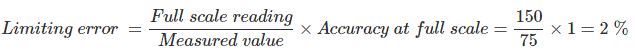09․ The total current I = I1 + I2 in a circuit is measured as I1 = 150 ± 1A , I2 = 250 ± 2A, where the limits of error are as standard deviations. I is measured as
(400 ± 1.5) A
(400 ± 1) A
(400 ± 3) A
(400 ± 2.24) A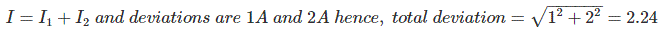10․ To measure 5 volts, if one selects a 0-100 volt range voltmeter which is accurate with in ± 1%, then the error in this measurement may be up to
± 1.5%
± 2.5%
± 7.5 %
± 20%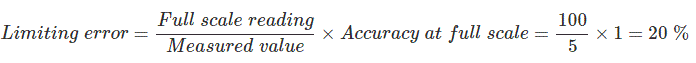<<<1516171819>>>